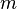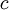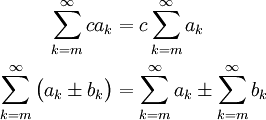# Sigma Notation Properties

Previous: Sigma Notation Terminology

Next: Changing Summation Limits

## The Properties

As one might expect, sigma notation follows the following properties.

Theorem: Sigma Notation Properties
Supposeandare functions of,is any integer, andis any real number. ThenFor these properties, we also require the infinite sums to exist. We will discuss what it means for an infinite sum to exist in the next lesson. These properties are easy to prove if we can write out the sums without the sigma notation.

## Simple Example

The infinite sumcan be written asCertainly, decomposing the combined sum in (1) into two sums in (2) does not give us a simpler representation. But this decomposition would allow us to more easily perform the convergence tests that we introduce in later lessons.

Previous: Sigma Notation Terminology

Next: Changing Summation Limits